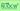# Optimum Design 1&2Lecturer Hideyuki AZEGAMI, Professor School of Informatics / Graduate School of Infomatics, 2021 Spring Graduate School of Informatics, Department of Complex Systems Science

### Goals of the Course

This course aims that student obtain the knowledge with which they understand systematically the practical world such as nature and society from an informatics viewpoint through simulation using methods in mathematical science, and with which they connect to problem solving.

### Objectives of the Course

#### Optimum Design 1

The purpose of this course is to help students acquire the basic concepts and principles in mathematical science with respect to optimum designs of systems. Moreover, in order to deal with function optimization problems in Optimum Design 2, students understand the concept of functional spaces that is extended from the concept of vector spaces.

#### Optimum Design 2

Subsequently to Optimum Design 1, students acquire the basic theories on existence of the solutions with respect to boundary value problems of partial differential equations and the principle of the numerical analysis. Using the knowledge, students understand the formulations of topology and shape optimization problems of continua and regular solutions of those problems.

### Course Content

#### Optimum Design 1

1. Introduction
2. Basics of Optimal Design
3. Basics of Optimization Theory
4. Basics of Mathematical Programming
5. Basics of Variational Principles and Functional Analysis

#### Optimum Design 2

1. Boundary Value Problems of Partial Differential Equations
2. Fundamentals of Numerical Analysis
3. Abstract Optimum Design Problem
4. Topology Optimization Problems of Density Variation Type
5. Shape Optimization Problems of Domain Variation Type

### Lecture materials

#### Optimum Design 1

1. Introduction

2. Basics of Optimal Design

3. Basics of Optimization Theory

4. Basics of Mathematical Programming

5. Basics of Variational Principles and Functional Analysis

#### Optimum Design 2

1. Boundary Value Problems of Partial Differential Equations

2. Fundamentals of Numerical Analysis

3. Abstract Optimum Design Problem

4. Topology Optimization Problems of Density Variation Type

5. Shape Optimization Problems of Domain Variation Type

#### Last updated

January 27, 2022

OCW website, and all course materials made public on this site,
may be used under the terms and conditions posted under "About OCW"
© 2020 Nagoya University Open Course Ware